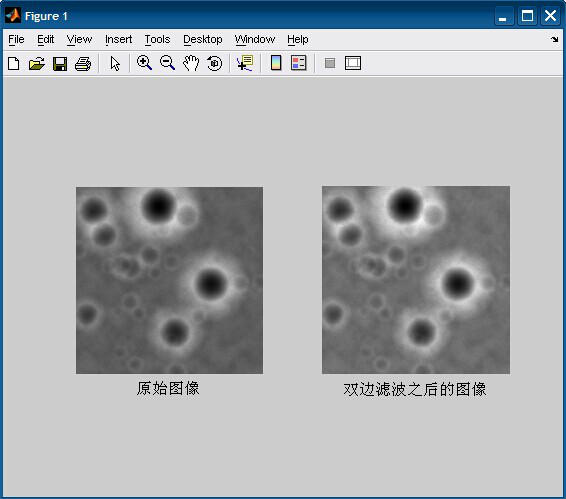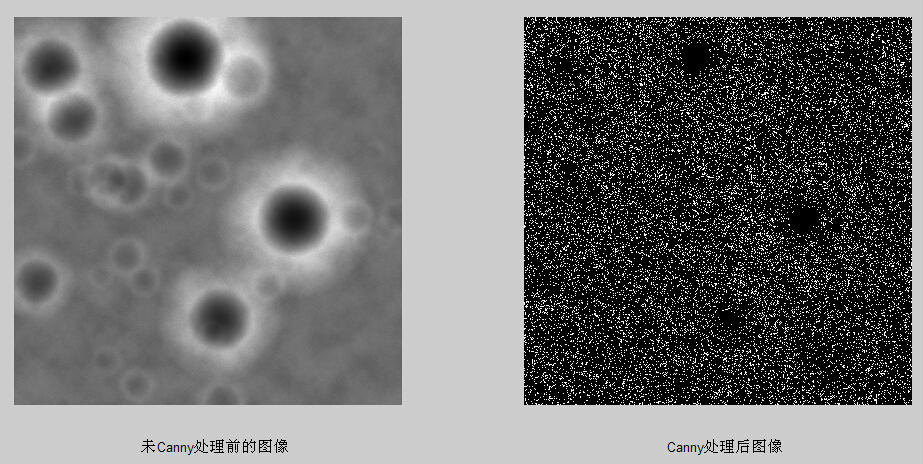• 三天的比赛结束了。今年的题比较变态，我已经被虐到无力吐槽了。。...没想到接触到图像处理是在数学建模的比赛。。。第二天和第三天啥也没干，就在死抠图像处理程序。最终在第三天晚上完美解决。
 三天的比赛结束了。今年的题比较变态，我已经被虐到无力吐槽了。。不过真是不到最后逼自己一把成不了事。最后一天突破了好多的困难。在最后的一小时里写完了论文，完成了全部的任务。    一开始报选修课的时候选matlab的原因是想学一下matlab的图像处理。然后课上一直讲的是基础。。没想到接触到图像处理是在数学建模的比赛中。。。第二天和第三天啥也没干，就在死抠图像处理程序。最终在第三天晚上完美解决。从第一天搜集的大量文献中总结筛选，最终决定了“原始照片 → 双边滤波处理（去噪）→ 切割分块取均值后重组图像 → Canny算子边缘检测 → 对比”的最佳线路。
双边滤波：程序：

1
2
3
4
5
6
7
8
9
10
11
12
13
14
15
16
17
18
19
20
21
22
23
24
25
26
27
28
29
30
31
32
33
34
35
36
37
38
39
40
41
42
43
44
45
46

%%%%%%%%图5.6程序%%%%%%%%%
clear all;
close all;
clc;
img=imread('附件3 距2400m处的数字高程图.tif');
% img=mat2gray(img);%2值化
 
%%%%%%%%%%第一个图：显示原始图像%%%%%%%%%%%%
subplot(121);
imshow(img);
title('原始图像','position',[1150,2600]);
%%%%%%%%%%%%%%%%%%%%%%%%%%%%%%%%%%
 
%%%%%%%%%%%%%%%%%%%%%双边滤波%%%%%%%%%%%%%%%%%%%%%%%
r=10;        %模板半径
[m n]=size(img);
imgn=zeros(m+2*r+1,n+2*r+1);
imgn(r+1:m+r,r+1:n+r)=img;
imgn(1:r,r+1:n+r)=img(1:r,1:n);                 %扩展上边界
imgn(1:m+r,n+r+1:n+2*r+1)=imgn(1:m+r,n:n+r);    %扩展右边界
imgn(m+r+1:m+2*r+1,r+1:n+2*r+1)=imgn(m:m+r,r+1:n+2*r+1);    %扩展下边界
imgn(1:m+2*r+1,1:r)=imgn(1:m+2*r+1,r+1:2*r);       %扩展左边界
sigma_d=2;
sigma_r=0.1;
[x,y] = meshgrid(-r:r,-r:r);
w1=exp(-(x.^2+y.^2)/(2*sigma_d^2));     %以距离作为自变量高斯滤波器
h=waitbar(0,'图像双边滤波中...');
for i=r+1:m+r
    for j=r+1:n+r

        w2=exp(-(imgn(i-r:i+r,j-r:j+r)-imgn(i,j)).^2/(2*sigma_r^2)); %以周围和当前像素灰度差值作为自变量的高斯滤波器
        w=w1.*w2;
        s=imgn(i-r:i+r,j-r:j+r).*w;
        imgn(i,j)=sum(sum(s))/sum(sum(w));
    end
    waitbar(i/m);
end
close(h)
%%%%%%%%%%%%%%%%%%%%%%%%%%%%%%%%%%%%%%%%%%%
 
%%%%%%%%%%%%%%第二个图：滤波后图像%%%%%%%%%%%%%%%%%
% figure;
img1=mat2gray(imgn(r+1:m+r,r+1:n+r));
subplot(122);
imshow(img1);
title('双边滤波之后的图像','position',[1160,2600]);
%%%%%%%%%%%%%%%%

Canny算子边缘检测程序：

1
2
3
4
5
6
7
8
9
10
11
12
13
14
15
16
17
18
19
20
21
22
23
24
25
26
27
28
29
30
31
32
33
34
35
36
37
38
39
40
41
42
43
44
45
46
47
48
49
50
51
52

%%%%%%%%%%%%%%%%%图5.7程序%%%%%%%%%%%%%%%%%%%%%%%%%%%
clear all;
close all;
clc;
img=imread('附件3 距2400m处的数字高程图.tif');
% img=mat2gray(img);%2值化
 
%%%%%%%%%%%%%%%%%%%%%双边滤波%%%%%%%%%%%%%%%%%%%%%%%
r=10;        %模板半径
[m n]=size(img);
imgn=zeros(m+2*r+1,n+2*r+1);
imgn(r+1:m+r,r+1:n+r)=img;
imgn(1:r,r+1:n+r)=img(1:r,1:n);                 %扩展上边界
imgn(1:m+r,n+r+1:n+2*r+1)=imgn(1:m+r,n:n+r);    %扩展右边界
imgn(m+r+1:m+2*r+1,r+1:n+2*r+1)=imgn(m:m+r,r+1:n+2*r+1);    %扩展下边界
imgn(1:m+2*r+1,1:r)=imgn(1:m+2*r+1,r+1:2*r);       %扩展左边界
sigma_d=2;
sigma_r=0.1;
[x,y] = meshgrid(-r:r,-r:r);
w1=exp(-(x.^2+y.^2)/(2*sigma_d^2));     %以距离作为自变量高斯滤波器
h=waitbar(0,'图像双边滤波中...');
for i=r+1:m+r
    for j=r+1:n+r

        w2=exp(-(imgn(i-r:i+r,j-r:j+r)-imgn(i,j)).^2/(2*sigma_r^2)); %以周围和当前像素灰度差值作为自变量的高斯滤波器
        w=w1.*w2;
        s=imgn(i-r:i+r,j-r:j+r).*w;
        imgn(i,j)=sum(sum(s))/sum(sum(w));
    end
    waitbar(i/m);
end
close(h)
%%%%%%%%%%%%%%%%%%%%%%%%%%%%%%%%%%%%%%%%%%%
 
%%%%%%%%%%%%%%第二个图：滤波后图像%%%%%%%%%%%%%%%%%
% figure;
img1=mat2gray(imgn(r+1:m+r,r+1:n+r));
subplot(121);
imshow(img1);
title('未Canny处理前的图像','position',[1200,2600])
%%%%%%%%%%%%%%%%%%%%%%%%%%%%%%%%%%%%%%%%%%%%%%%%
 
 
%%第四个图：第二个图滤波后图像的canny边缘检测处理结果%%
% canny边缘检测
h2=waitbar(0,'绘制双边滤波之后的canny图...');
img4 = edge(img1,'canny');  % 调用canny函数
waitbar(3);
subplot(122)
imshow(img4);  % 显示分割后的图像，即梯度图像
title('Canny处理后图像','position',[1200,2600])
close(h2);
%%%%%%%%%%%%%%%%%%%%%%%%%%%%%%%%%%%%%%%%%%%%%%%%%%%

由上图可以看出，处理效果并不好。我们进行切割分块取均值后再还原图像的方法处理后再进行Canny算子边缘检测。
图片：程序：

1
2
3
4
5
6
7
8
9
10
11
12
13
14
15
16
17
18
19
20
21
22
23
24
25
26
27
28
29
30
31
32
33
34
35
36
37
38
39
40
41
42
43
44
45
46
47
48
49
50
51
52
53
54
55
56
57
58
59
60
61
62
63
64
65
66
67
68
69
70
71
72
73
74

%%%%%%%%%%%%%%%%图5.8程序%%%%%%%%%%%%%%%%%%%%%%%%%%%%
clear all;
close all;
clc;
img=imread('附件3 距2400m处的数字高程图.tif');
 
 
%%%%%%%%%%%%%%%%%%%%%双边滤波%%%%%%%%%%%%%%%%%%%%%%%
r=10;        %模板半径
[m n]=size(img);
imgn=zeros(m+2*r+1,n+2*r+1);
imgn(r+1:m+r,r+1:n+r)=img;
imgn(1:r,r+1:n+r)=img(1:r,1:n);                 %扩展上边界
imgn(1:m+r,n+r+1:n+2*r+1)=imgn(1:m+r,n:n+r);    %扩展右边界
imgn(m+r+1:m+2*r+1,r+1:n+2*r+1)=imgn(m:m+r,r+1:n+2*r+1);    %扩展下边界
imgn(1:m+2*r+1,1:r)=imgn(1:m+2*r+1,r+1:2*r);       %扩展左边界
sigma_d=2;
sigma_r=0.1;
[x,y] = meshgrid(-r:r,-r:r);
w1=exp(-(x.^2+y.^2)/(2*sigma_d^2));     %以距离作为自变量高斯滤波器
h=waitbar(0,'图像双边滤波中...');
for i=r+1:m+r
    for j=r+1:n+r

        w2=exp(-(imgn(i-r:i+r,j-r:j+r)-imgn(i,j)).^2/(2*sigma_r^2)); %以周围和当前像素灰度差值作为自变量的高斯滤波器
        w=w1.*w2;
        s=imgn(i-r:i+r,j-r:j+r).*w;
        imgn(i,j)=sum(sum(s))/sum(sum(w));
    end
    waitbar(i/m);
end
close(h)
 
%%%%%%%%%%%%%%第二个图：滤波后图像%%%%%%%%%%%%%%%%%
img1=mat2gray(imgn(r+1:m+r,r+1:n+r));
 
 
%%%%%%%%%%滤波后图像高程值分块单位化并求均值%%%%%%%%%
a=zeros(575);
b=zeros(575);
c=zeros(575);
d=zeros(575);
H=waitbar(0,'滤波后图像高程值分块单位化并求均值...');%粗壁障，采用4*4计算单位。
for i=1:1:575;
    for j=1:1:575;
        a(j,i)=img1(4*j-1,4*i-1);
        b(j,i)=img1(4*j-1,4*i);
        c(j,i)=img1(4*j,4*i-1);
        d(j,i)=img1(4*j,4*i);
        a1=a(j,i);b1=b(j,i);c1=c(j,i);d1=d(j,i);
        img2(j,i)=(a1+b1+c1+d1)/16;% 取平均数之后
    end
    waitbar(i/575);
end
close(H);
%%%%%%%%%%%%%%%%%%%%%%%%%%%%%%%%%%%%%%%%%%%%%%%%%
 
 
%%%%第三个图：滤波后图像高程值分块单位化后均值排列图%%%%%%
% figure(1);
% imagesc(img1);
% figure(2);
subplot(121)
imshow(img2);
title('未Canny处理前的图像','position',[287,630])
 
 
 
%%%%%%第五个图：第三个图的canny边缘检测处理结果%%%%%%%
% canny边缘检测
img5 = edge(img2,'canny');  % 调用canny函数
subplot(122);
imshow(img5);  % 显示分割后的图像，即梯度图像
title('Canny处理后图像','position',[287,635])
%%%%%%%%%%%%%%%%%%%%%%%%%%%%%%%%%%%%%%%%%%%%%%%%%%%


展开全文• 数学建模文件（含图像处理算法）包括了一些常用算法的代码啊
• 数学建模–数字图像处理–亮度变换与空间滤波在数学建模竞赛，还存在一类大的模块就是数字图像处理相关算法的使用以及图像的显示。最近正好也在学数字图像处理这一课程，基于两者利用Matlab编程给出一些示例数学建模 图像处理 算法
• http://blog.sina.com.cn/s/blog_62e14ece01014m3c.html
• matlab 数学建模 图像处理 2017-10-22 00:43:21
I = imread('ellipse.BMP'); Ig = rgb2gray(I); imshow(Ig); hold on conicP = ginput(15); plot(conicP(:,1)', conicP(:,2)', 'xr'); %drawing sample points a0 = [1 1 1 1 1 1];...f = @(a,x)a(1)*x(:,1).^2+a(2数学建模 图像处理
• 作者:July 二零一一年一月二十九日 本文参考： I、 细数二十世纪最伟大的十大算法 [译者：本人July] ...1、此数学建模十大算法依据网上的一份榜单而写，本文对此十大算法作一一简单介绍。 这只是一份榜单而已，数学建模 代码
• 数学建模中python常用数学计算库还不全SciPy函数库在NumPy库的基础上增加了众多的数学、科学以及工程计算常用的库函数。例如线性代数、常微分方程数值求解、信号处理、图像处理、稀疏矩阵等等。由于其涉及的领域...数学建模 数学计算
• 数学建模常用软件 2019-05-09 11:24:58
我参加过的数学建模比赛很多，除了本校的两次数学建模（二三等）外，全国数学建模（省二），亚太数学建模（s），ICM/MCM（M），五一建模联赛，电工杯（最近正在准备），之前错过mathorcup，有点遗憾。到2019年暑假前...
• 如何入门参与数学建模 2019-05-21 13:23:26
数学建模感想 纪念逝去的大学数学建模：两次校赛，两次国赛，两次美赛，一次电工杯。从大一下学期组队到现在，大三下学期，时间飞逝，我的大学建模生涯也告一段落。感谢建模路上帮助过我的学长和学姐们，滴水之恩当...大学生数学建模
• MATLAB数学建模 2019-10-08 14:51:46matlab图像处理 bp神经网络matlab实例
• 频域变换为了快速有效地对图像进行处理和分析，常需要将原定义在图像空间的图像以某种形式转换到频域空间，并利用频域空间的特有性质方便地进行一定的加工，最后再转换回图像空间，得到需要的效果。数学建模 图像处理
• 数学建模问题分析 2020-04-13 23:00:34
数据处理 1）插值拟合：对数据补全和基本趋势分析 对数据补全和基本趋势分析（根据某个未知函数（或已知但难于求解的函数）的几个已知数据点求出变化规律和特征相似的近似曲线的过程。） 插值：求过已知有限个数据点...数学建模
• 数学建模的概念: 所谓的数学建模就是使用数学框架解决实际应用问题,数学建模在解决问题的同时能够培养 1. 数学思维能力， ...数学建模中的典型方法 蒙特卡罗算法 数据拟合、参数估计、插值等数据处理算法数学建模 算法
• 数学建模到人工智能 ...为什么要把数学建模与当今火热的人工智能放在一起？...首先，数学建模在字面上可以分解成数学+建模...数学建模往往是没有对与错，只有"更好"（better），就好像让你评价两个苹果哪个更好...程序员 人工智能 数学建模
• 数学建模算法与应用》方法概述序言：学习司守奎《数学建模算法与应用》第2版过程，给出一个内容的提纲，希望可以在回忆复习时快速建立知识结构。包含了数学建模所需用到的一些方法和常见问题类型。 其中前半...数学建模 算法
• 数学建模常.模型及其MATLAB等软件实现 讲师：清风 更多视频请在B站或优酷关注UP主：数学建模学习交流 十大模型，涉及近三十种算法 2 / 94 更多视频请在B站或优酷关注UP主：数学建模学习交流 为什么录制这门课程？ ...数学建模
• 图像去噪是非常基础也是非常必要的研究，去噪常常在更高级的图像处理之前进行，是图像处理的基础。可惜的是，目前去噪算法并没有很好的解决方案，实际应用，更多的是在效果和运算复杂度之间求得一个平衡，再一次...算法
• 本书涵盖了很多同类型书籍较少涉及的新算法和热点技术，主要内容包括时间序列、支持向量机、偏最小二乘面归分析、现代优化算法、数字图像处理、综合评价与决策方法、预测方法以及数学建模经典算法等内容。...
• 本书涵盖了很多同类型书籍较少涉及的新算法和热点技术，主要内容包括时间序列、支持向量机、偏最小二乘面归分析、现代优化算法、数字图像处理、综合评价与决策方法、预测方法以及数学建模经典算法等内容。...
...